Anúncio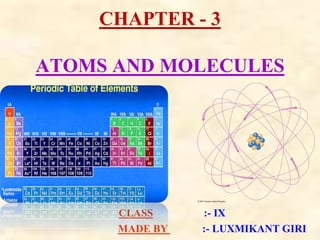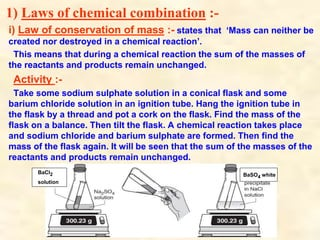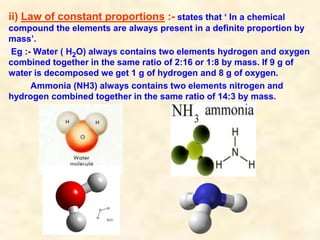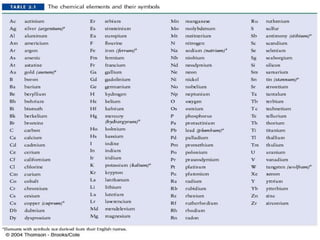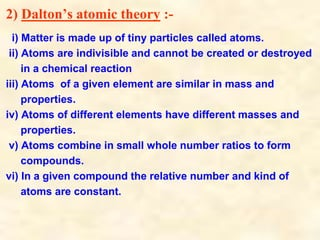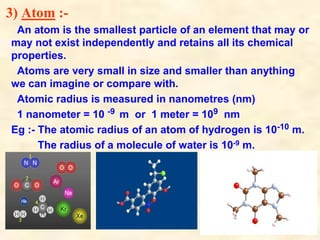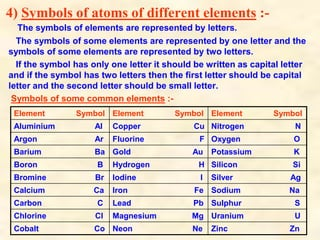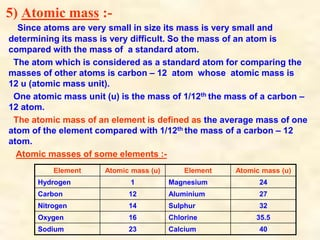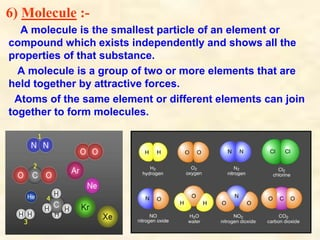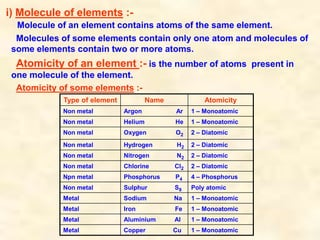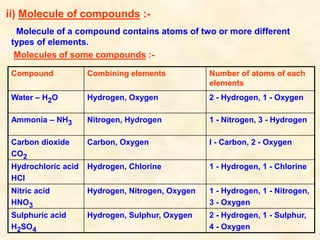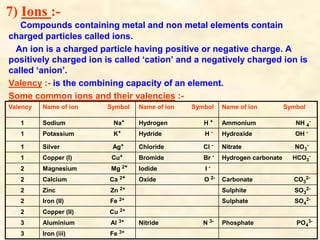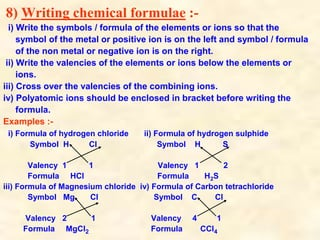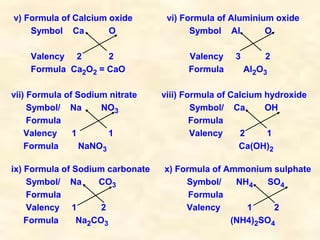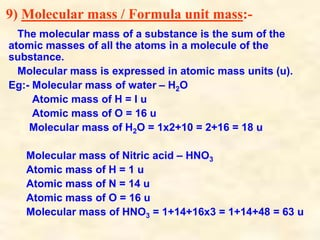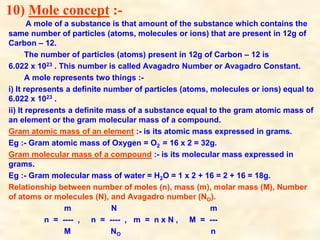1 de 17
Anúncio

### CHP%203%20ATOMS%20AND%20MOLECULES%20PPTX.pptx

1. CHAPTER - 3 ATOMS AND MOLECULES CLASS :- IX MADE BY :- LUXMIKANT GIRI
2. 1) Laws of chemical combination :- i) Law of conservation of mass :- states that ‘Mass can neither be created nor destroyed in a chemical reaction’. This means that during a chemical reaction the sum of the masses of the reactants and products remain unchanged. Activity :- Take some sodium sulphate solution in a conical flask and some barium chloride solution in an ignition tube. Hang the ignition tube in the flask by a thread and pot a cork on the flask. Find the mass of the flask on a balance. Then tilt the flask. A chemical reaction takes place and sodium chloride and barium sulphate are formed. Then find the mass of the flask again. It will be seen that the sum of the masses of the reactants and products remain unchanged. BaCl2 solution BaSO4 white
3. ii) Law of constant proportions :- states that ‘ In a chemical compound the elements are always present in a definite proportion by mass’. Eg :- Water ( H2O) always contains two elements hydrogen and oxygen combined together in the same ratio of 2:16 or 1:8 by mass. If 9 g of water is decomposed we get 1 g of hydrogen and 8 g of oxygen. Ammonia (NH3) always contains two elements nitrogen and hydrogen combined together in the same ratio of 14:3 by mass.
4. 2) Dalton’s atomic theory :- i) Matter is made up of tiny particles called atoms. ii) Atoms are indivisible and cannot be created or destroyed in a chemical reaction iii) Atoms of a given element are similar in mass and properties. iv) Atoms of different elements have different masses and properties. v) Atoms combine in small whole number ratios to form compounds. vi) In a given compound the relative number and kind of atoms are constant.
5. 3) Atom :- An atom is the smallest particle of an element that may or may not exist independently and retains all its chemical properties. Atoms are very small in size and smaller than anything we can imagine or compare with. Atomic radius is measured in nanometres (nm) 1 nanometer = 10 -9 m or 1 meter = 109 nm Eg :- The atomic radius of an atom of hydrogen is 10-10 m. The radius of a molecule of water is 10-9 m.
6. 4) Symbols of atoms of different elements :- The symbols of elements are represented by letters. The symbols of some elements are represented by one letter and the symbols of some elements are represented by two letters. If the symbol has only one letter it should be written as capital letter and if the symbol has two letters then the first letter should be capital letter and the second letter should be small letter. Symbols of some common elements :- Element Symbol Element Symbol Element Symbol Aluminium Al Copper Cu Nitrogen N Argon Ar Fluorine F Oxygen O Barium Ba Gold Au Potassium K Boron B Hydrogen H Silicon Si Bromine Br Iodine I Silver Ag Calcium Ca Iron Fe Sodium Na Carbon C Lead Pb Sulphur S Chlorine Cl Magnesium Mg Uranium U Cobalt Co Neon Ne Zinc Zn
7. 5) Atomic mass :- Since atoms are very small in size its mass is very small and determining its mass is very difficult. So the mass of an atom is compared with the mass of a standard atom. The atom which is considered as a standard atom for comparing the masses of other atoms is carbon – 12 atom whose atomic mass is 12 u (atomic mass unit). One atomic mass unit (u) is the mass of 1/12th the mass of a carbon – 12 atom. The atomic mass of an element is defined as the average mass of one atom of the element compared with 1/12th the mass of a carbon – 12 atom. Atomic masses of some elements :- Element Atomic mass (u) Element Atomic mass (u) Hydrogen 1 Magnesium 24 Carbon 12 Aluminium 27 Nitrogen 14 Sulphur 32 Oxygen 16 Chlorine 35.5 Sodium 23 Calcium 40
8. 6) Molecule :- A molecule is the smallest particle of an element or compound which exists independently and shows all the properties of that substance. A molecule is a group of two or more elements that are held together by attractive forces. Atoms of the same element or different elements can join together to form molecules.
9. i) Molecule of elements :- Molecule of an element contains atoms of the same element. Molecules of some elements contain only one atom and molecules of some elements contain two or more atoms. Atomicity of an element :- is the number of atoms present in one molecule of the element. Atomicity of some elements :- Type of element Name Atomicity Non metal Argon Ar 1 – Monoatomic Non metal Helium He 1 – Monoatomic Non metal Oxygen O2 2 – Diatomic Non metal Hydrogen H2 2 – Diatomic Non metal Nitrogen N2 2 – Diatomic Non metal Chlorine Cl2 2 – Diatomic Npn metal Phosphorus P4 4 – Phosphorus Non metal Sulphur S8 Poly atomic Metal Sodium Na 1 – Monoatomic Metal Iron Fe 1 – Monoatomic Metal Aluminium Al 1 – Monoatomic Metal Copper Cu 1 – Monoatomic
10. ii) Molecule of compounds :- Molecule of a compound contains atoms of two or more different types of elements. Molecules of some compounds :- Compound Combining elements Number of atoms of each elements Water – H2O Hydrogen, Oxygen 2 - Hydrogen, 1 - Oxygen Ammonia – NH3 Nitrogen, Hydrogen 1 - Nitrogen, 3 - Hydrogen Carbon dioxide CO2 Carbon, Oxygen I - Carbon, 2 - Oxygen Hydrochloric acid HCl Hydrogen, Chlorine 1 - Hydrogen, 1 - Chlorine Nitric acid HNO3 Hydrogen, Nitrogen, Oxygen 1 - Hydrogen, 1 - Nitrogen, 3 - Oxygen Sulphuric acid H2SO4 Hydrogen, Sulphur, Oxygen 2 - Hydrogen, 1 - Sulphur, 4 - Oxygen
11. 7) Ions :- Compounds containing metal and non metal elements contain charged particles called ions. An ion is a charged particle having positive or negative charge. A positively charged ion is called ‘cation’ and a negatively charged ion is called ‘anion’. Valency :- is the combining capacity of an element. Some common ions and their valencies :- Valency Name of ion Symbol Name of ion Symbol Name of ion Symbol 1 Sodium Na+ Hydrogen H + Ammonium NH 4 - 1 Potassium K+ Hydride H - Hydroxide OH - 1 Silver Ag+ Chloride Cl - Nitrate NO3 - 1 Copper (I) Cu+ Bromide Br - Hydrogen carbonate HCO3 - 2 Magnesium Mg 2+ Iodide I - 2 Calcium Ca 2+ Oxide O 2- Carbonate CO3 2- 2 Zinc Zn 2+ Sulphite SO3 2- 2 Iron (II) Fe 2+ Sulphate SO4 2- 2 Copper (II) Cu 2+ 3 Aluminium Al 3+ Nitride N 3- Phosphate PO4 3- 3 Iron (iii) Fe 3+
12. 8) Writing chemical formulae :- i) Write the symbols / formula of the elements or ions so that the symbol of the metal or positive ion is on the left and symbol / formula of the non metal or negative ion is on the right. ii) Write the valencies of the elements or ions below the elements or ions. iii) Cross over the valencies of the combining ions. iv) Polyatomic ions should be enclosed in bracket before writing the formula. Examples :- i) Formula of hydrogen chloride ii) Formula of hydrogen sulphide Symbol H Cl Symbol H S Valency 1 1 Valency 1 2 Formula HCl Formula H2S iii) Formula of Magnesium chloride iv) Formula of Carbon tetrachloride Symbol Mg Cl Symbol C Cl Valency 2 1 Valency 4 1 Formula MgCl2 Formula CCl4
13. v) Formula of Calcium oxide vi) Formula of Aluminium oxide Symbol Ca O Symbol Al O Valency 2 2 Valency 3 2 Formula Ca2O2 = CaO Formula Al2O3 vii) Formula of Sodium nitrate viii) Formula of Calcium hydroxide Symbol/ Na NO3 Symbol/ Ca OH Formula Formula Valency 1 1 Valency 2 1 Formula NaNO3 Ca(OH)2 ix) Formula of Sodium carbonate x) Formula of Ammonium sulphate Symbol/ Na CO3 Symbol/ NH4 SO4 Formula Formula Valency 1 2 Valency 1 2 Formula Na2CO3 (NH4)2SO4
14. 9) Molecular mass / Formula unit mass:- The molecular mass of a substance is the sum of the atomic masses of all the atoms in a molecule of the substance. Molecular mass is expressed in atomic mass units (u). Eg:- Molecular mass of water – H2O Atomic mass of H = I u Atomic mass of O = 16 u Molecular mass of H2O = 1x2+10 = 2+16 = 18 u Molecular mass of Nitric acid – HNO3 Atomic mass of H = 1 u Atomic mass of N = 14 u Atomic mass of O = 16 u Molecular mass of HNO3 = 1+14+16x3 = 1+14+48 = 63 u
15. 10) Mole concept :- A mole of a substance is that amount of the substance which contains the same number of particles (atoms, molecules or ions) that are present in 12g of Carbon – 12. The number of particles (atoms) present in 12g of Carbon – 12 is 6.022 x 1023 . This number is called Avagadro Number or Avagadro Constant. A mole represents two things :- i) It represents a definite number of particles (atoms, molecules or ions) equal to 6.022 x 1023 . ii) It represents a definite mass of a substance equal to the gram atomic mass of an element or the gram molecular mass of a compound. Gram atomic mass of an element :- is its atomic mass expressed in grams. Eg :- Gram atomic mass of Oxygen = O2 = 16 x 2 = 32g. Gram molecular mass of a compound :- is its molecular mass expressed in grams. Eg :- Gram molecular mass of water = H2O = 1 x 2 + 16 = 2 + 16 = 18g. Relationship between number of moles (n), mass (m), molar mass (M), Number of atoms or molecules (N), and Avagadro number (NO). m N m n = ---- , n = ---- , m = n x N , M = --- M NO n
Anúncio